## Example Questions

### Example Question #1 : Sales Tax

If a sweater has a total cost of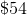after tax, what is the cost of the sweater before tax, if the sales tax is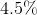?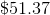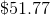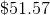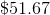Explanation:

The total cost of the sweater is the original cost plus a 4.5% tax, which can be expressed in the equation: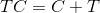Where TC is the total cost, C is the original cost, and T is the tax.  Now, we will plug in the information we know.  Since the original cost of the sweater is unknown, we will use the variable x.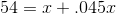.045 is the decimal expression of 4.5%.

Now, solve for x.  Consolidate the x variable.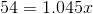Divide both sides by 1.045.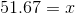is the cost of the sweater before tax.

### Example Question #2 : Sales Tax

A cake order cost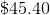before tax.  If the tax rate is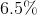, what is the price of the cake after tax is applied?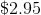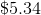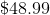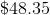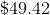Explanation:

Remember: For all percentage problems, you need to convert your percentage to a decimal before working on your equation.

You can solve this problem one of two ways.  The first way, which is less efficient, is to multiply the original amount by the tax rate and then add that to the original: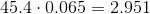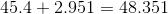or:The easier way to do this is to multiply your original amount byplus the tax rate.  (This does the addition for you in one step.)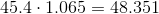or:Notice that your problem is asking for the total new cost, not the amount of tax to be added!

### Example Question #3 : Sales Tax

A can of rare beans costs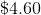after tax and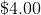before.  What is the tax rate on this item?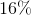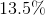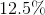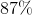Explanation:

Remember: For all percentage problems, you need to convert your percentage to a decimal before working on your equation.

The easiest way to solve this problem is to convert it into a clear sentence.  The question is asking, "What percentage ofis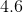?"  This is the same thing as writing: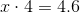(Recall, "of" becomes multiplication and "is" becomes equals.)

Solve this by dividing both sides by: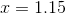This means thatis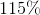of.  In other words, it is aincrease to.  This is the tax rate.

### Example Question #4 : Sales Tax

How much tax is charged on a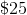umbrella if its tax rate is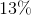?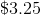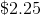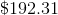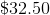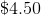Explanation:

Remember: For all percentage problems, you need to convert your percentage to a decimal before working on your equation.

This problem is very simple, for you only have to compute the tax amount in dollars—not the complete price.

Therefore, you merely need to multiply the original price by the tax rate.

This gives you: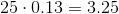### Example Question #5 : Sales Tax

In the past year, the price for a bottle of water decreased bywhile the sales tax on the bottle of water is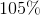of the original rate. What percentage does the tax increase/decrease by compared to last year?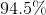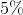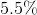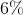Explanation:

For this problem, we are given no numbers to work with. Therefore, we can substitute a number of our choosing into the problem. For simplicities sake, the number chosen will be $100. If the price of water decreased by 10%, that would mean that the new price for water if the old price was$100 is $90. Because tax increased by 5%, that would mean that for a bottle of water, if we assume the old tax rate was 10%, the new tax rate is 10.5%. This would mean that if previously, if you paid 10% tax for a$100 bottle of water, you would pay $10 in taxes. Now, you would pay 10.5% tax for a$90 bottle of water meaning you would pay \$9.45 in tax.

This problem asks you for the percentage the tax changes by, therefore in order to find the percent change, we divide the new tax by the old tax,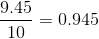or 94.5%. This means that the amount of tax paid has decreased by 5.5% compared to the following year.

### Example Question #6 : Sales Tax

A supermarket sells apples for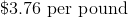. If you buy more than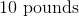of apples at once, the supermarket will discount the price by.  How much will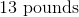of apples cost? Round to the nearest cent.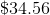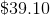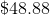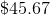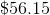In this problem, we are asked to find the price ofof apples.  The original price of apples is stated to be.  That would mean thatof apples would cost. Because the supermarket gives adiscount if you buy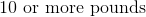of apples at once,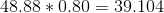.  Rounded to the nearest cent, 13 pounds of apples would cost.